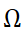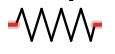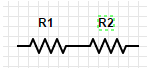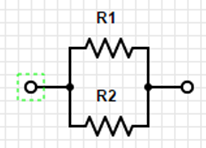# 1.02 Relationship between voltage and current: resistors

Art of Electronics 2nd Edition p 4-7

What you MUST learn

Resistance (represented in formulas as “R”) measured in Ohms which is represented using the symbol:Resistance is the relationship between Current (I) and Voltage (R).

This relationship is defined by Ohm’s law:  R = V/I.

Circuit diagrams represent resistors asResistors in Series: (Always bigger)R= R1 + R2Resistors in Parallel: (Always smaller)

R = (R1*R2)/(R1+R2)

Shortcut #1:

A large resistor in series with a small resistor has the resistance of the larger one, roughly.

A large resistor in parallel with a  small resistor has the resistance of the smaller one, roughly.

Try to develop an intuitive sense of the voltage and current in the parts of the circuit.  Do not try to calculate to be extremely precise because the precision of the components will make this pointless.  Also, good circuit design should be tolerant of variations.

Power:

P=IV

Using Ohm’s law you get:

P=(I^2)R

P=(V^2)/R

Things good to learn

The inverse of resistance is conductance (represented by “G” in formulas)  G=1/R

Restatement of Ohm’s law:  I=GV

Conductance is measured in siemenssiemens is also known as mho (ohm spelled backwards)